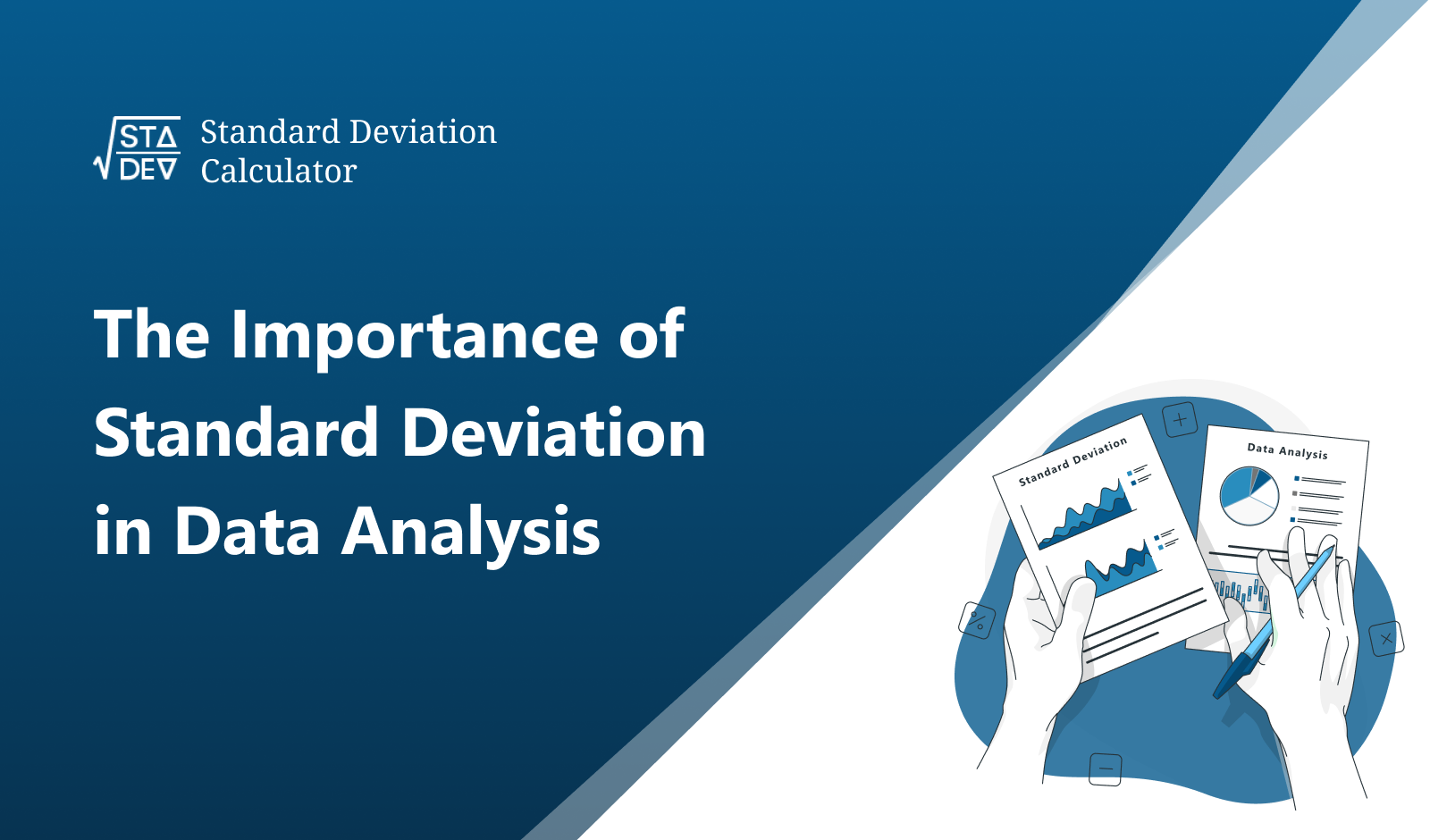Jun 7, 2023
The Importance of Standard Deviation in Data AnalysisIn the contemporary digital world, where every bit of information matters, the significance of data analysis cannot be overlooked. One key element in data analysis is the measure of dispersion, the standard deviation.

Understanding the standard deviation and its importance in data analysis offers valuable insights and fosters informed decision-making.

This article will delve into the value of the standard deviation, its applications, and how using a tool such as the "Standard Deviation Calculator" can simplify calculations.

## Standard Deviation: A Fundamental Metric in Statistics.

Standard deviation is a statistical concept that quantifies the variation or dispersion of a set of values. This essential measure represents how spread out the numbers in a data set are. If the standard deviation is small, it signifies that the data points tend to be close to the mean.

Conversely, a high standard deviation indicates that the values are spread out over a wider range.

The role of standard deviation becomes increasingly important when dealing with large datasets, complex analytics, and statistical modeling. It is a critical factor in many areas such as finance, psychology, physics, and business management.

## The Role of Standard Deviation in Data Analysis:

The standard deviation plays a vital role in data analysis, it is used for:

• Risk analysis
• Quality control
• Predictive Analytics
• Descriptive analytics

### Risk Analysis:

In finance, standard deviation is used to measure risk and volatility. A higher standard deviation signifies higher risk and potential for variability in returns.

### Quality Control:

Industries use standard deviation for quality assurance. Products falling outside the standard deviation range may indicate a quality issue.

### Predictive Analytics:

In predictive modeling and data science, standard deviation helps identify outliers and anomalies in the data.

### Descriptive Statistics:

Standard deviation, along with mean and median, offers a summary of a dataset. This trio provides a comprehensive view of the data distribution.

## Simplifying Calculations with the Standard Deviation Calculator:

Conducting standard deviation calculations manually can be challenging, especially with large data sets. This is where the Standard Deviation Calculator comes in handy. This intuitive, user-friendly tool simplifies your calculations, saving time and effort. With just a few clicks, you can easily calculate the standard deviation for any given set of data.

Q1: What does a high standard deviation indicate?

A: A high standard deviation indicates that the data points are spread out over a wider range, away from the mean. It signifies high variability in the dataset.

Q2: How can I calculate standard deviation easily?

A: You can use an online tool like the Standard Deviation Calculator for a quick, accurate, and easy calculation.

Q3: Is standard deviation applicable in business scenarios?

A: Absolutely! Standard deviation plays a significant role in financial risk assessment, quality control, market research, and many other business scenarios.

## Wrapping Up:

Understanding and using the standard deviation measure effectively is critical to successful data analysis. Tools like the Standard Deviation Calculator can significantly simplify this process. So, leverage this essential statistical measure to unlock the full potential of your data and make informed decisions.

Remember, in the age of data, knowledge is power, and standard deviation is one of the keys to this power.